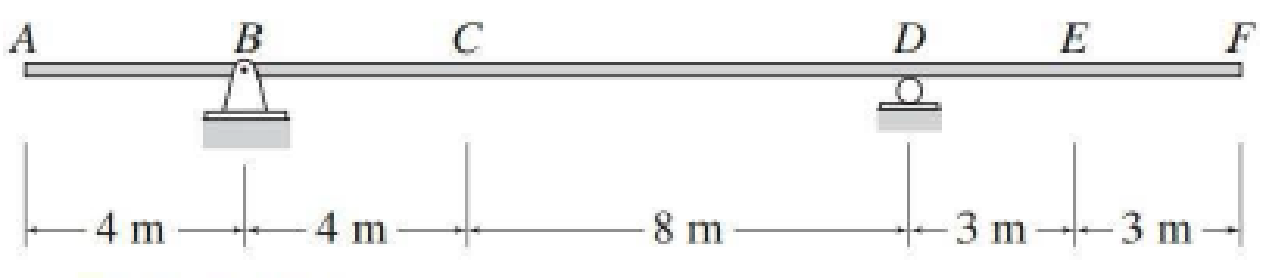# Draw the influence lines for the shear and bending moment at point E of the beam shown in Fig. P8.10. FIG. P8.10, P8.11

#### Solutions

Chapter
Section
Chapter 8, Problem 11P
Textbook Problem
23 views

## Draw the influence lines for the shear and bending moment at point E of the beam shown in Fig. P8.10.FIG. P8.10, P8.11

To determine

Draw the influence lines for the shear and bending moment at point E.

### Explanation of Solution

Calculation:

Find the equation of shear force at just right E (0x3m).

Apply 1 kN load at just right of E.

Consider section EF.

Sketch the free body diagram of the section EF as shown in Figure 1.

Refer Figure 1.

Apply equilibrium equation of forces.

Consider upward force as positive (+) and downward force as negative ().

ΣFy=0

SE1=0SE=1

Find the equation of shear force at just left of E (3mx22m).

Apply 1 kN load at just left of E.

Consider section EF.

Sketch the free body diagram of the section EF as shown in Figure 2.

Refer Figure 2.

Apply equilibrium equation of forces.

Consider upward force as positive (+) and downward force as negative ().

ΣFy=0

SE=0

Thus, the equations of the influence line for SE as follows,

SE=1, 0x3m        (1)

SE=0, 3mx22m        (2)

Find the shear force at various points of x using the Equations (1) and (2) and summarize the value as in Table 1.

 Position x (m) SE (kN/kN) F 0 1 E 3+ 1 E 3− 0 D 6 0 C 14 0 B 18 0 A 22 0

Draw the influence lines for the shear force at point E using Table 1 as shown in Figure 4

### Still sussing out bartleby?

Check out a sample textbook solution.

See a sample solution

#### The Solution to Your Study Problems

Bartleby provides explanations to thousands of textbook problems written by our experts, many with advanced degrees!

Get Started

Find more solutions based on key concepts
Calculate the efficacy for the following lights: (a) Sylvania accent LED light, which uses 2 watts and produces...

Engineering Fundamentals: An Introduction to Engineering (MindTap Course List)

What are data warehousing and data mining? How do businesses use these tools?

Systems Analysis and Design (Shelly Cashman Series) (MindTap Course List)

What is a disjoint subtype? Give an example.

Database Systems: Design, Implementation, & Management

What does NFPA stand for?

Precision Machining Technology (MindTap Course List)

True or False? The Hyundai Sonata Hybrid uses BAS and ISAD systems.

Automotive Technology: A Systems Approach (MindTap Course List)

A _____ contains data about each file in the database and each field in those files. (510) a. query b. form c. ...

Enhanced Discovering Computers 2017 (Shelly Cashman Series) (MindTap Course List)

If your motherboard supports ECC DDR3 memory, can you substitute non-ECC DDR3 memory?

A+ Guide to Hardware (Standalone Book) (MindTap Course List)